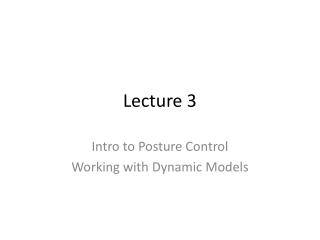DownloadDownload PresentationLecture 3

# Lecture 3

Télécharger la présentation## Lecture 3

- - - - - - - - - - - - - - - - - - - - - - - - - - - E N D - - - - - - - - - - - - - - - - - - - - - - - - - - -
##### Presentation Transcript

1. Lecture 3 Intro to Posture Control Working with Dynamic Models

2. The need for Posture Controllers Joint Controllers (PD) Posture Controllers Compensates for non-idealities: Vibration Compliance in joints and limbs Changes in mass distribution Force/torque disturbances Changes to the mathematical model • Generate voltage to produce desired torque/speed • Move links to follow generated path

3. Landing Controllers What must be controlled? How it’s done Apply torque at ankle during landing Deflect knee joint to create virtual shock absorber Extend or retract landing leg to change cycle timing Add delay to walking cycle until landing is achieved, • Angular momentum • Landing Impact Force • Landing timing • Uneven foot placement

4. Keeping the Torso Vertical • Tilted floors introduce pitch/roll error • Need independent orientation data • Correct with simple PI control to ankles/knees

5. Impact Force Reduction • Prescribe knee deflection as ideal mass-spring-damper • Determine maximum allowable force • Determine total landing time dt • Apply 2 constraints to determine ideal k, c

6. Angular Momentum compensation • Desired angular momentum • Compensate by applying angular impulse

7. Dynamic Modeling

8. Types of Modeling Tools AutoLev ADAMS GUI based dynamics simulation Uses predefined joints and rigid bodies to build system Simulates system based on a specified input Difficult to interface with external software packages • Script-based • Can produce Inverse Kinematics, Equations of motion, and PID control • Relies on external solver (Matlab or C)

9. What do we model? Should be included Can be sometimes be ignored Flexure of structure under low stress / low frequency excitement Lump small masses Ignore small inertia contributions • Large and heavy bodies • Joints with motors • Compliant joints • virtual spring and damper

10. Simplification of Hubo Actual Hubo Simplified model (10 DOF) TSO RUL LUL RLL LLL RFT LFT

11. Joint design for Inverse Kinematics • Need to be able to describe limbs in terms of end position • Ball joints at hip and ankle require Euler angles • Use gimbal joints to simplify description

12. Modeling Procedure • Define rigid bodies (geometry, mass, inertia) • Define any extra reference frames • Describe geometric relationships between bodies/frames • Define p, v, a for each mass center • Define θ,ω, α for each body • Define applied forces/torques • Use Kane’s method to produce equations of motion

13. Validate the model Zero Input Behavior Static Equilibrium Multiple inverted pendulum has equilibria at: qi=0, all links vertical q1=pi, qi=0, (i=2..n) (All links hanging) • Intuitive behavior: • Collapse due to gravity • Swing infinitely with no applied damping • Settle with damping at joints Equilibria w/ static Torque • Apply torque at each joint to balance weight T3(t) T2(t) T1(t)

14. Add PD Control to joints Proportional Control Derivative Control • Define error qerri • Compare to reference ri • Increase proportional gain to improve trajectory tracking • Define error qerri • Compare to reference ri • Increase derivative gain to damp overshoot

15. Inputs and Initial conditions Initial Conditions Input from Inverse Kinematics # ref inputs = # Independent system DOF Use IK to translate trajectories into ri (see IK example ) • Initial angles and velocities must be valid • AL: Solve initial conditions using nonlinear eq. solver • (See IC example)

16. Boundaries • Work envelope limits possible trajectories • Choose desired trajectory within work envelope

17. Model Update: Taking a step Double Support (3D) Single support (3D) Necessary conditions at switch time T PD(T)=PS(T) VD(T)=VS(T) Very simple - No collision dynamics

18. Model Update: Foot Landing Single Support (3D) Double support (3D) Necessary conditions @ T PS(T)=PD(T) VS(T)≠VD(T) HS(T)=HD(T) Vi,n=0